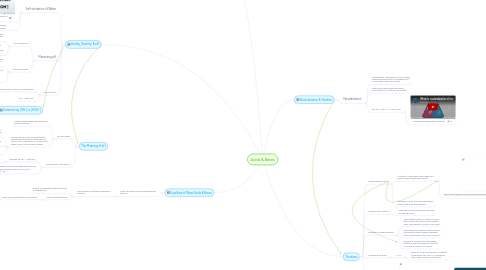# Acids & BasesCinda Murray
Get Started. It's FreeAcids & Bases## 2. The Meaning of pH

### 2.1. pH and Acidity

2.1.1. Neutral: When acidity and basicity are exactly balanced

2.1.2. Danish chemist Soren Sorensen (1909) discovered focusing not on the value of [H3O+] but on the power of 10 that arises when [H3O+] is in scientific notation

2.1.2.1. Proposed using the negative of the power of 10 of [H3O+] as the index of acidity and basicity. He called this measure the pH.

2.1.2.2. The letters p and H represent power of hydrogen

2.1.2.3. pH values at Specified [H3O+]

### 2.2. Calculating pH from [H3O+]

2.2.1. Equation for: pH = -log[H3O+]

2.2.1.1. pH of 0 = Very Acidic, pH of 14 = Very Alkaline, pH of 7 = Neutral

2.2.2. Equation for calculating the hydronium ion concentration from the pH: [H3O+] = 10^-pH

## 3. Equilibria of Weak Acids & Bases

### 3.1. Acids can donate protons and bases pick them up

3.1.1. Acid-Ionization constant is equilibrium constant

3.1.1.1. Buffers contain equal weak acid and its conjugate base

3.1.1.2. buffers stabalize the Ph

3.1.1.2.1. foods and household items are buffered

## 4. Acidity, Basicity, & pH

### 4.1. Self-ionization of Water

4.1.1. Equilibrium between water & the ions it forms

4.1.2. -Concentrations of hydronium ions in a solution expresses its: Acidity -Concentrations of hydroxide ions in a solution expresses its: Basicity

### 4.2. Measuring pH

4.2.1. Using indicatiors

4.2.1.1. Indications- compound that can reversibly change color depending on the pH of the solution or the chemical change

4.2.1.2. Ex. of indications- thymol blue ( yellow in pH 3 & 8 but blue in 10+), lithimus (natural). Universal indicators change colors for the entire scale.

4.2.2. Using a pH meter

4.2.2.1. pH meter- electronic instrument w/ a probe that is dipped in a solution of an unknown pH.

4.2.2.2. The probe has 2 electrodes, 1 is sensitive to the hydronium ion. An electrical voltage develops between the electrodes, and it measures the voltage. It converts the measurement into a pH.

### 4.3. Calculating pH

4.3.1. Find the H30+ and OH- concentrations

4.3.1.1. Kw = [H30+] * [OH-]

4.3.1.2. Kw = 1.00 x 10^-14

4.3.2. pH = -log[H30+]

## 5. What are Acids & Bases?

### 5.1. Acids

5.1.1. Electrolytes that conduct electrical current well in solutions of water.

5.1.1.1. Strong acid- an acid that ionizes completely in a solvent.

5.1.1.2. Weak acid- an acid that releases few hydrogen ions in aqueous solution. (when dissolved in H2O, only fraction of its molecules are ionized)

5.1.2. Characterized by the presence of H3O+.

5.1.3. Aqueous solutions of acids react with many metals.

### 5.3. Bronsted-Lowry Classification

5.3.1. Acid is any compound that can transfer a proton

5.3.2. A base is any compound that can receive a proton

## 6. Neutralization & Titration

### 6.1. Neutralization

6.1.1. Neutralization- the reaction of the ions that characterize acids (H3O+) and bases (OH-) to form water molecules and salt

6.1.2. When you combine equal amounts of H3O+(aq) and OH-(aq) they form water.

6.1.3. EX. HCL + NaCl ----> NaCl +H20

6.1.3.1. Neutralization explanation/ example

## 7. Titrations

### 7.1. The Equivalence Point

7.1.1. A Titration Curve graph results when pH is plotted against the titreant volume

7.1.1.1. http://img.sparknotes.com/figures/3/3a5994498f24d59f5d5d762b40844a2a/sasb.gif

7.1.2. A titration is exact only if the equivalance point can be accurately deteced

### 7.2. Carrying Out a Titration

7.2.1. When the pH level changes the Indicatior will change colors

### 7.3. Selecting a Suitable Indicator

7.3.1. The Transition Range, or stage, is at a pH level in which the indication will change color, with respect to the pH of the tirant

7.3.2. The end point in with the instant in which the indicator stops changes changeing colors, with respect to the level of the pH

7.3.3. Example of a strong acid, strong base titration is that the equivalnce point will occur at a pH level of 7, or netual.

### 7.4. Calculating Titrations

7.4.1. n=cV

7.4.1.1. where (c) is the concentration, in respect to moles per liter, and (V), in respect to liters, is the volume of the solution

### 7.5. Understanding titrations

7.5.1. Titration introduction